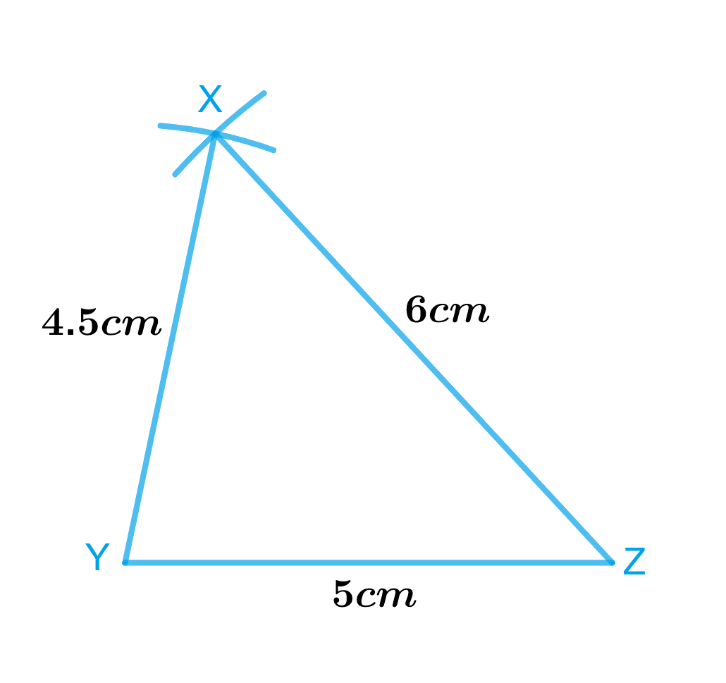# Ex.10.2 Q1 Practical Geometry Solution- NCERT Maths Class 7

Go back to  'Ex.10.2'

## Question

Construct $$ΔXYZ$$ in which $$XY =4.5\,\rm{cm,}$$ $$YZ = 5\,\rm{cm}$$ and $$ZX =6\,\rm{cm}.$$

Video Solution
Practical Geometry
Ex 10.2 | Question 1

## Text Solution

What is known?

Lengths of sides of a triangle $$XYZ$$ are $$XY =4.5\,\rm{cm,}$$ $$YZ = 5\,\rm{cm}$$ and $$ZX =6\,\rm{cm}.$$

To construct:

A triangle $$XYZ$$ in which $$XY =4.5\,\rm{cm},$$  $$YZ = 5\,\rm{cm}$$  and $$ZX =6\,\rm{cm}.$$

Reasoning:

We will draw a rough sketch of $$ΔXYZ$$ with the given measure. This will help us in deciding how to proceed. Then follow the steps given below.

Steps:Steps of construction

1. Draw a line segment $$YZ$$ of length $$5$$ $$\rm{cm.}$$
2. From $$Y$$, point $$X$$ is at a distance of $$4.5$$ $$\rm{cm}.$$. So, with $$Y$$ as centre, draw an arc of radius $$4.5$$ $$\rm{cm}.$$ (now $$X$$ will be somewhere on this arc & our job is to find where exactly $$X$$ is).
3. From $$Z$$, point $$X$$ is at a distance of $$6$$ $$\rm{cm}.$$. So, with $$Z$$ as centre, draw an arc of radius $$6$$ $$\rm{cm}.$$ (now $$X$$ will be somewhere on this arc, we have to fix it).
4. $$X$$ has to be on both the arcs drawn. So, it is the point of intersection of arcs. Mark the point of intersection of arcs as $$X$$. Join $$XY$$ and $$XZ.$$

Thus, $$XYZ$$ is the required triangle.

Learn from the best math teachers and top your exams

• Live one on one classroom and doubt clearing
• Practice worksheets in and after class for conceptual clarity
• Personalized curriculum to keep up with school